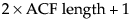This example analyzes the gas furnace data (seriesJ.jmp) from Box and Jenkins. To begin the analysis, select Input Gas Rate as the Input List and Output CO2 as the Y, Time Series. The launch dialog should appear as in Series J Launch Dialog.
Series J Launch Dialog
When you click OK, the report in Series J Report appears.
Series J Report
Each report section has its own set of commands. For the output (top) series, the commands are accessible from the red triangle on the outermost outline bar (Transfer Function Analysis). For the input (bottom) series, the red triangle is located on the inner outline bar (Input Series: Input Gas Rate).
Output and Input Series Menus shows these two command sets. Note their organization. Both start with a Graph command. The next set of commands are for exploration. The third set is for model building. The fourth set includes functions that control the platform.
Output and Input Series Menus
Both parts give basic diagnostics, including the sample mean (Mean), sample standard deviation (Std), and series length (N).
Basic diagnostics also include the autocorrelation and partial autocorrelation functions, as well as the Ljung-Box Q-statistic and p-values, found under the Time Series Basic Diagnostics outline node.
The Cross Correlation command adds a cross-correlation plot to the report. The length of the plot is twice that of an autocorrelation plot, or.
Cross Correlation Plot
To prewhiten the input series, select the Prewhitening command. This brings up a dialog similar to the ARIMA dialog where you specify a stochastic model for the input series. For our SeriesJ example, we use an ARMA(2,2) prewhitening model, as shown in Prewhitening Dialog.
Prewhitening Dialog
Click Estimate to reveal the Prewhitening plot.
Yt denotes the output series
X1 to Xm denote m input series
et represents the noise series
X1, td1 indicates the series X1 is indexed by t with a d1-step lag
μ represents the mean level of the model
ϕ(B) and θ(B) represent autoregressive and moving average polynomials from an ARIMA model
ωk(B) and δk(B) represent numerator and denominator factors (or polynomials) for individual transfer functions, with k representing an index for the 1 to m individual inputs.
Select Transfer Function to bring up the model specification dialog.
Transfer Function Specification Dialog
specifies whether μ is zero or not.
Series J Transfer Function Reports